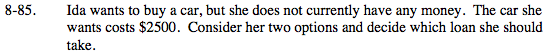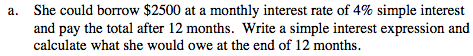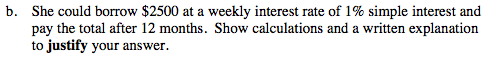### Home > MC2 > Chapter 8 > Lesson 8.2.6 > Problem8-85

8-85.Use the equation for simple interest from the Math Notes box in this lesson.
Interest = Prt where:
P = Principal
r = rate
t = time

Interest = ($2500)(0.04)(12 months) ($2500)(0.04) = $100 dollars per month in interest ($100)(12 months) = $1200 interest over 12 months How much total money does Ida owe under this option if her borrowed amount is$2500 and her interest would be $1200?Use the same interest formula from part (a). Interest = (principal)(interest rate)(weeks in a year) There are 52 weeks in a year. Ida owes$3800 with this option. Be sure to show how to get this answer and explain which one of the two loan options is better and why.